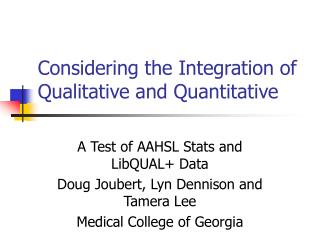# Considering the Integration of Qualitative and Quantitative - PowerPoint PPT PresentationDownload PresentationConsidering the Integration of Qualitative and Quantitative

Considering the Integration of Qualitative and Quantitative
Download Presentation## Considering the Integration of Qualitative and Quantitative

- - - - - - - - - - - - - - - - - - - - - - - - - - - E N D - - - - - - - - - - - - - - - - - - - - - - - - - - -
##### Presentation Transcript

1. Considering the Integration of Qualitative and Quantitative A Test of AAHSL Stats and LibQUAL+ Data Doug Joubert, Lyn Dennison and Tamera Lee Medical College of Georgia

2. Local Questions • Do any patterns exist between AAHSL Annual Stats and LibQUAL+ data? • Required combining the data from both data sets into a common SPSS file

3. Local Questions • AAHSL Data Transformation • Examined the Expenditures Summary data from AAHSL Annual Statistics • Transformed and recoded the AAHSL data to accommodate for missing scores • For example, with data import, SPSS needed to understand that “M” was a “system missing” value

4. Local Questions • AAHSL Data Transformation • Coded AAHSL variables: • Personnel expendituresperexp • Total Collection Expenditures tocex • Total Recurring Expenditures toreex • Capital Budget capbud • Total Annual Expenditures toanex

5. Local Questions • AAHSL Data Transformation • Combined the information from both data sets into a single SPSS data file • Grouped data by a common variable: instID • Merged the two files via SPSS

6. Local Questions • SPSS Data Transformation • Identified stats for the Affect of Service Dimension • LibQUAL+ already created variables for person level subscales • Specifically, the minimum, desired, and perceived means for each of the 2002 Dimensions • Computed the means of Service Affect Dimension for each participating institution

7. Local Questions • SPSS Data Transformation • To compute the means of Service of Affect Dimension for institutions we used the following variables from LibQUAL • aavgmin1 • aavgdes1 • aavgper1

8. Local Questions • SPSS Data Transformation • Computing the means of Service of Affect Dimension for each institution allowed us to compute a mean gap for each institution • This was accomplished in much the same way as computing the LibQUAL+ gap • Average perceived – average minimum = average gap (by institution)

9. Local Questions • SPSS Data Transformation • Having the average gap (by institution) allowed us to look at the “relationship” between it and the total annual expenditures (AAHSL)

10. Local Questions • SPSS Data Transformation • Having the average gap (by institution) also allowed us to transform the gap score into a T-score (Norm Table) • As discussed Cook et al., T-scores allow one to examine individuals scores in relation to scores of peer insitutions1

11. Norms based on Affect of Service Gap

12. Local Questions • Questions for further exploration • The scatter plot visually reveals no relationship between gap score and Total Annual Expenditures

13. Local Questions • Questions for further exploration • Q 1: What valid statistical method may be used to measure correlation with the gap score? • For example: Spearman rank-order, Pearson correlation, or Linear Regression • Q 2: How do we develop percentile ranks based on T-scores (norms) in any number of questions and dimensions?

14. References • Cook, C., Heath, H., and Thompson, B. Score Norms for Improving Library Service Quality: A LibQUAL+ Study. portal: Libraries and the Academy, vol. 2, no. 1, pp. 13-26. (2002)